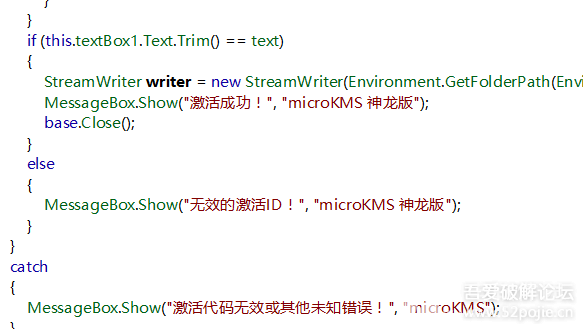KMS激活win10/win11和office2021/2019 MicroKMS神龙版 v21.12.08无广告版

目前MicroKMS神龙版能激活win10、win8/8.1、win7以及server2008/2012/2016等系统版本，其中包括：企业版、专业版（零售、VOL、WMC）、家庭版、教育版、核心版、中文版（OEM预装系统版本）、单语言版等，同时支持激活office 2010/2013/2016 Proplus、Visio 201private void button4_Click(object sender, EventArgs e)
{
try
{
string text = this.textBox3.Text;
string str2 = "";
for (int i = text.Length - 1; i >= 0; i--)
{
str2 = str2 + text.ToString();
}
text = str2;
for (int j = 0; j < text.Length; j++)
{
if (text[j] == 'A')
{
text = text.Replace(text[j], '0');
}
else if (text[j] == 'B')
{
text = text.Replace(text[j], '9');
}
else if (text[j] == 'C')
{
text = text.Replace(text[j], '8');
}
else if (text[j] == 'D')
{
text = text.Replace(text[j], '7');
}
else if (text[j] == 'E')
{
text = text.Replace(text[j], '6');
}
else if (text[j] == 'F')
{
text = text.Replace(text[j], '5');
}
else if (text[j] == 'Q')
{
text = text.Replace(text[j], '9');
}
else if (text[j] == 'R')
{
text = text.Replace(text[j], '8');
}
else if (text[j] == 'S')
{
text = text.Replace(text[j], '7');
}
else if (text[j] == 'T')
{
text = text.Replace(text[j], '6');
}
else if (text[j] == 'U')
{
text = text.Replace(text[j], '5');
}
else if (text[j] == 'V')
{
text = text.Replace(text[j], '4');
}
else if (text[j] == 'W')
{
text = text.Replace(text[j], '3');
}
else if (text[j] == 'X')
{
text = text.Replace(text[j], '2');
}
else if (text[j] == 'Y')
{
text = text.Replace(text[j], '1');
}
else if (text[j] == 'Z')
{
text = text.Replace(text[j], '0');
}
}
if (this.textBox1.Text.Trim() == text)
{
StreamWriter writer = new StreamWriter(Environment.GetFolderPath(Environment.SpecialFolder.Windows) + @"\sysinfo.dll", true, Encoding.GetEncoding("utf-8"));
MessageBox.Show("激活成功！", "microKMS 神龙版");
base.Close();
}
else
{
MessageBox.Show("无效的激活ID！", "microKMS 神龙版");
}
}
catch
{
MessageBox.Show("激活代码无效或其他未知错误！", "microKMS");
}
}
string text = this.textBox3.Text;这句话就是获取机器码，然后它写了一个循环把机器码做了一个倒序，后面一串if else就是把字母换成数字，最后把得到的数字序列和输入的激活ID比较，判断是否激活成功。### 更新日志

v19.01.03更新说明
1、修复了激活office存在的部分bug问题
2、修复了激活windows server版本出现异常的问题
v18.12.25更新说明
1、新增了对win10 N系列版本的激活支持
2、优化了office、visio、project的激活机制

v18.10.06更新说明
1、新增对win10 企业版 LTSC 2019的激活支持
2、新增对windows server 2019的激活支持
3、从microkms中剥离了亦美win10优化工具
-增加了对office2019、visio2019、project2019的激活支持
-修复了一些错误提示
-增加了一些win10优化功能

### 版本说明

-去除原版注册、弹网页渣行为；
-去除自动更新，让你一个版本可以用很久；
-去除界面所有无关内容，仅保留激活选项；